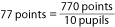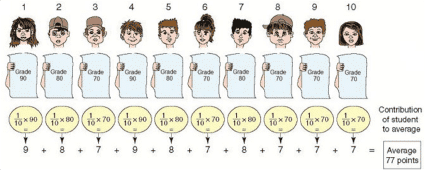# Meaning of Average

An average is a single number that results from a calculation. It indicates the approximate middle of a group of data of similar characteristics that are measured using the same units. The group of data can represent the grades of children in 1st grade in school, the height of 10 year-old children, etc. A more exact definition of the term “average” will be presented later in the chapter.

How to calculate an average

We will present three methods of calculating an average using as an example the grades of ten 4th grade students on an arithmetic test.

First method: Add up the grades, and divide by the number of pupils

Table 3.1 lists the pupils’ grades on the test.

Table 3.1

 Order of Pupils(alphabetized by name) Grade(in points) Pupil no. 1 90 points Pupil no. 2 80 points Pupil no. 3 70 point Pupil no. 4 90 points Pupil no. 5 80 points Pupil no. 6 70 points Pupil no. 7 80 points Pupil no. 8 70 points Pupil no. 9 70 points Pupil no. 10 70 points Total Grades 770 points AverageIn order to calculate the average, we add up all the pupils’ grades. The result is 770 points, which we divide by the number of pupils to get 77 points. 77 points is the average grade.

A More Exact Definition of the Average:

We will refer to the total of the group’s grade points (770 points) as “the original total of the group’s grades”. The average is the number that, had all the marks been identical and equal to it, then their total would be the same as the original total (of the group’s marks).

This means that 77 (the average) times 10 = 770 points.

Second method: Using the contribution of each pupil compared to the average

As we will immediately see, every pupil contributes some number of points to the average. Two factors affect the size of the contribution:

1. His grade – the higher the grade, the greater the student’s contribution to the average.

2. The student’s relative proportion in the class (explanation later).

The expression relative proportion indicates the pupil’s scores in relation to the total number of pupils in the class:

• In a class of 10 pupils, each pupil constitutes 1/10 of the class, or 10%.

• In a class of 2 pupils, each pupil constitutes 1/2 of the class, or 50%.

• In a class of 1 pupil, each pupil constitutes the entirety of the class, or 100%.

The larger the pupil’s relative proportion, the greater their contribution to the average.

Instead of the expression relative proportion, economists use the term weight. From now on, we will use the latter term (in most cases).

The following illustration displays the contribution of each pupil to the average. The illustration is divided into two parts:

• Part A shows each of the 10 pupils with the grades he received.

• Part B shows the contribution of each pupil to the average, and how it was calculated.

It can be seen in the illustration that pupil no.1 contributes 9 points to the average. His contribution is obtained by multiplying his mark (90 points) by his weight in the class (10%). Pupil no. 2 contributes 8 points to the average (his mark is 80 points, and his weight is 10%), and so on, up through the tenth pupil.

Illustration 3.1Third method: Using groups of pupils with the same grade

This method is the most popular, and is also the simplest in many cases. For purposes of the calculation, we group the pupils of the class according to the grades they received.

Illustration 3.2It can be seen in the illustration that two factors affect the size of each group’s contribution:

• The group’s grade – the higher the grade, the greater the group’s contribution to the average.

• The group’s weight – the group’s weight is the total weight of all the pupils in the group.

In our example, each pupil’s weight is 10% (therefore, the weight of 3 pupils is 30%, and the weight of 5 pupils is 50%).

It can be seen in the illustration that:

• Group 1 contributes 18 points to the average.

• Group 2 contributes 24 points to the average.

• Group 3 contributes 35 points to the average.

The three groups contribute a total of 77 points, which is the average.

Organizing the Data in a Table

Table 3.2

 Numbering the Groups Observation Data of the Group (the Grades) Number of Items in Each Group The Group’s Weight The Group’s Contribution to the Average 1 2 3 4 2 = 5 x 4 Group 1 90 pts. 2 20% 18 pts. Group 2 80 pts. 3 30% 24 pts. Group 3 70 pts. 5 50% 35 pts. Total 10 100% 77 pts. (the average)